CIM Created Edited

# [Feature & Edit] Using Sweep Translate

## Question

I would like to arrange the objects along a path according to the rules.

The Sweep Translate feature allows the user to perform these tasks.

The Sweep Translate feature moves and copies target objects along any selected path.
The example below shows this feature being used to copy the selected target object along the semi-circle path with R=10.0m.

Pattern Spacing & User Defined

ㆍThe target object (Solid) is copied at intervals of 5m along the length of the defined arc path with R=10.0m.
(Figure. 1)
ㆍThe target object (Solid) is copied at intervals of 2m, 4m, 6m, 8m, and 10m along the defined length of the arc path
with R=10.0m. (Figure. 2)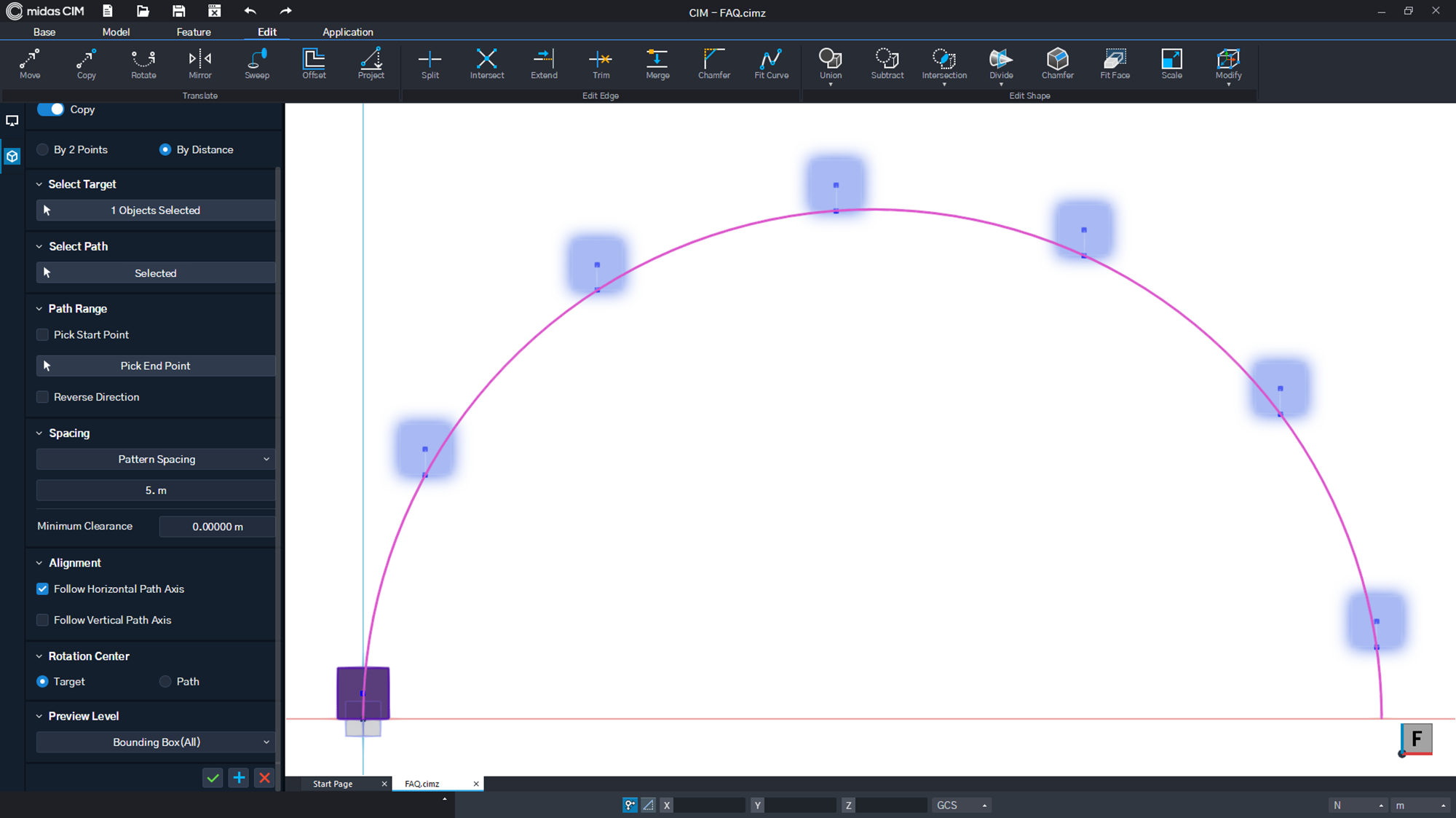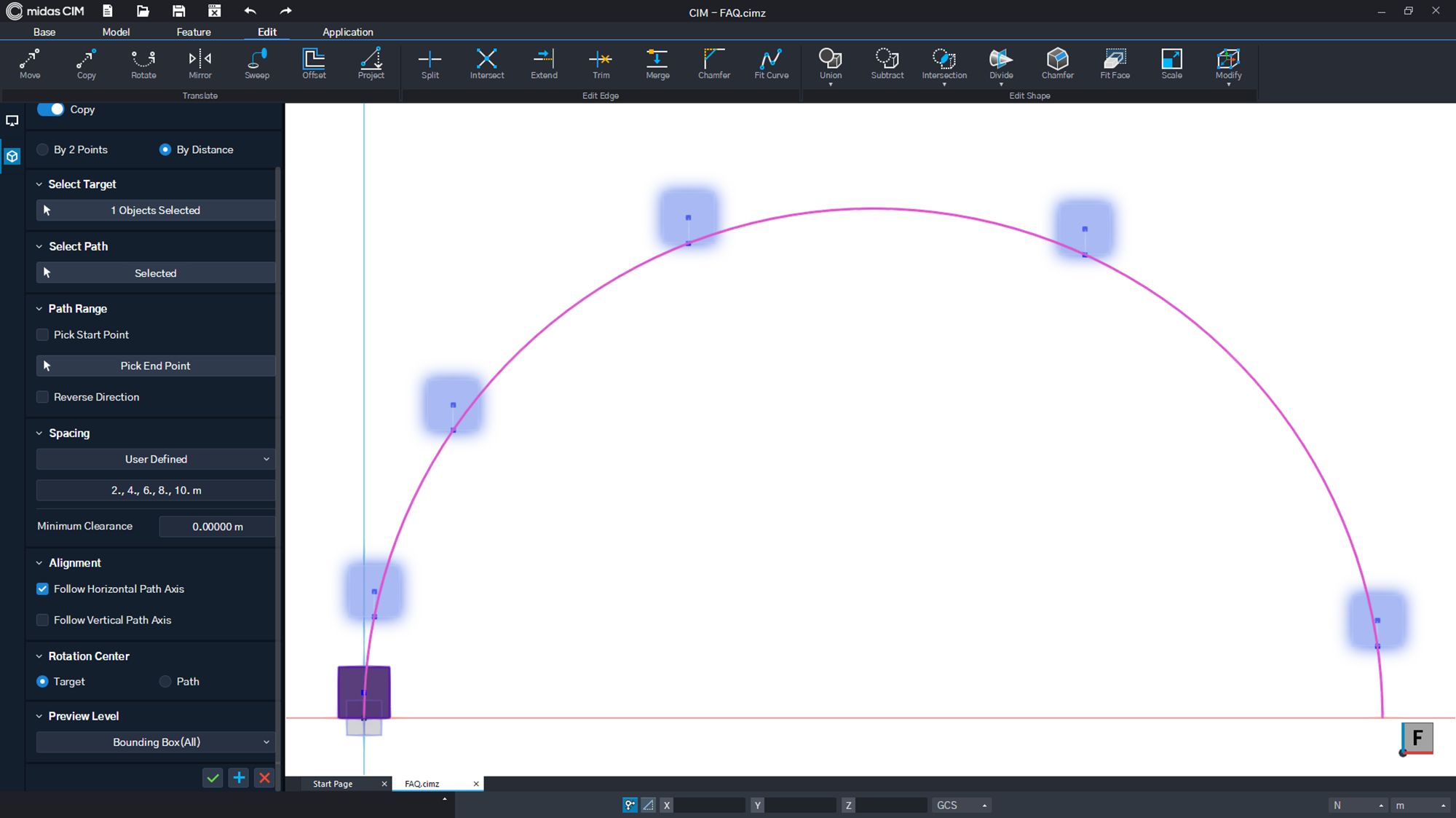Figure. 1 Pattern Spacing (5.0m) Figure. 2 User Defined (2, 4, 6, 8, 10m)

Uniform Divisions
ㆍThe target object (Solid) is copied to points that divide the defined arc path (R=10.0m) into five equal intervals.
(Figure. 3)
ㆍMin. Clearance : This is used to offset the end point by 1.0m and then copy the target object to points that
divide the defined arc path (R=10.0m) into five equal intervals.(Figure. 4)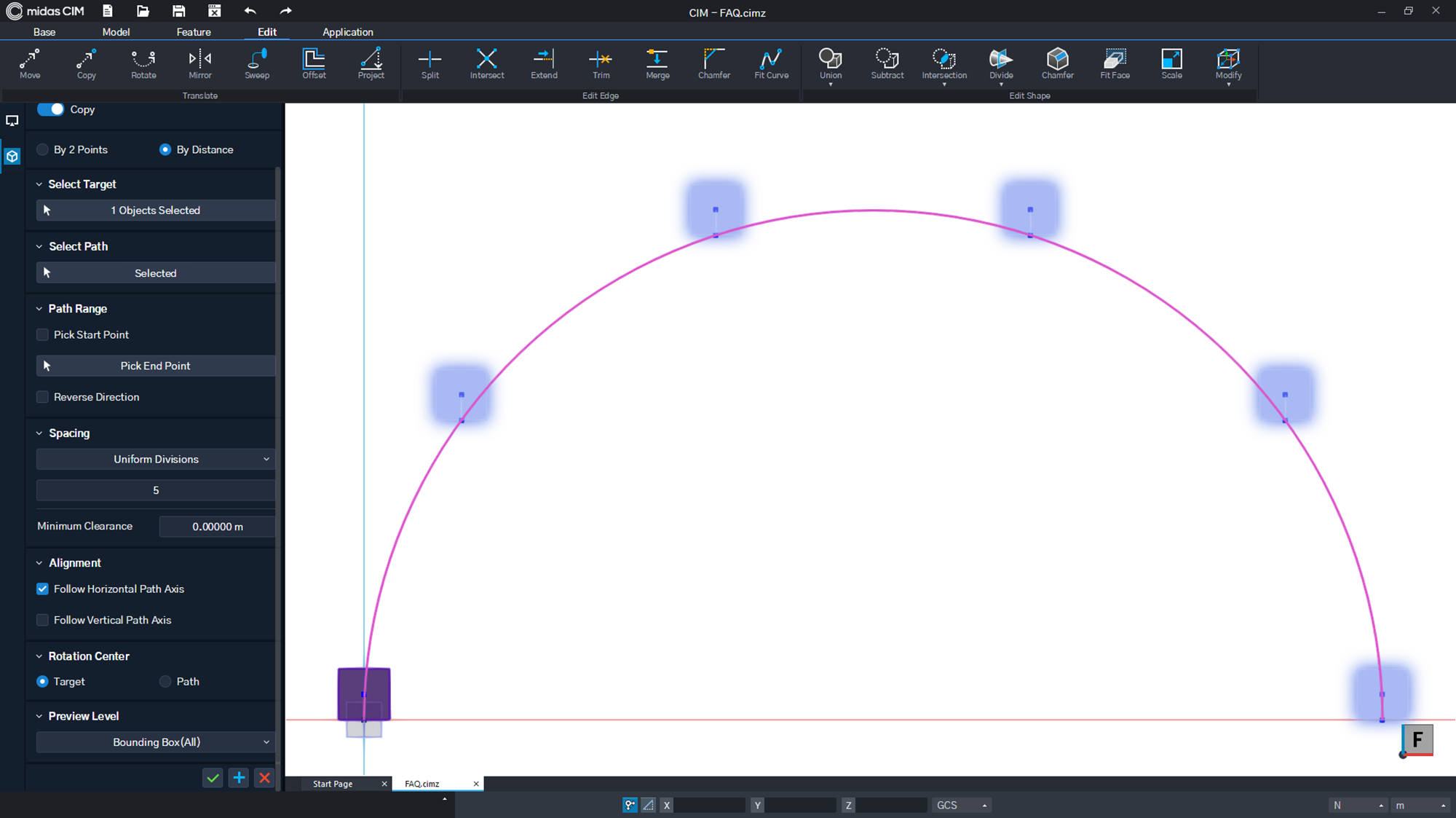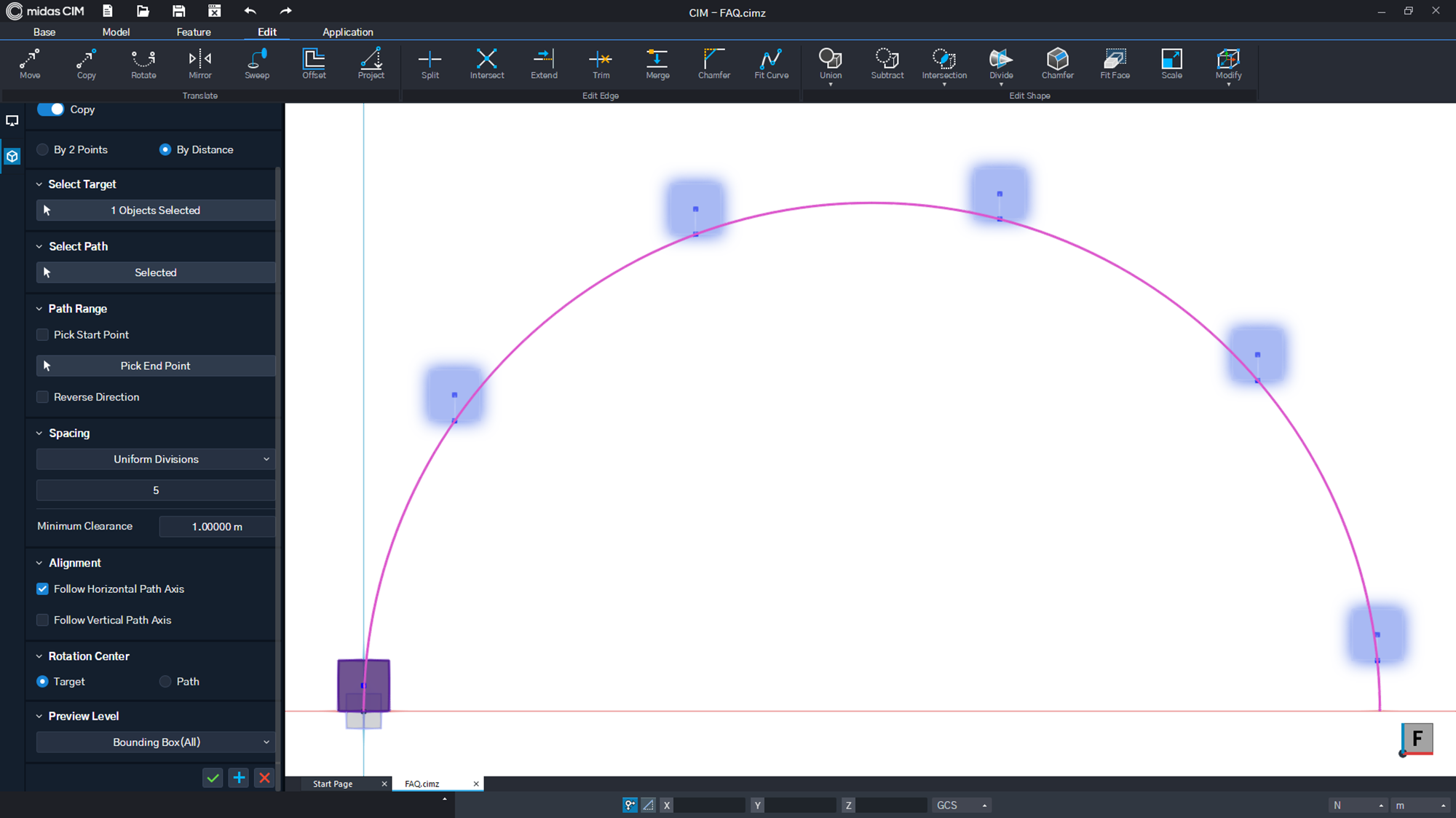Figure. 3 Uniform Divisions (Min. Clearance : 0.0m) Figure. 4 Uniform Divisions (Min. Clearance : 1.0m)

Uniform Max. Spacing
ㆍThe target object (Solid) is copied at an interval smaller than the Uniform Max.
Spacing of 10m along the defined arc path (R=10.0m) (Figure. 5)
ㆍSince the circumference of the semi-circle is πr=31.4m, the target object will be copied four times when a value
smaller than 10m is applied. (Figure. 6)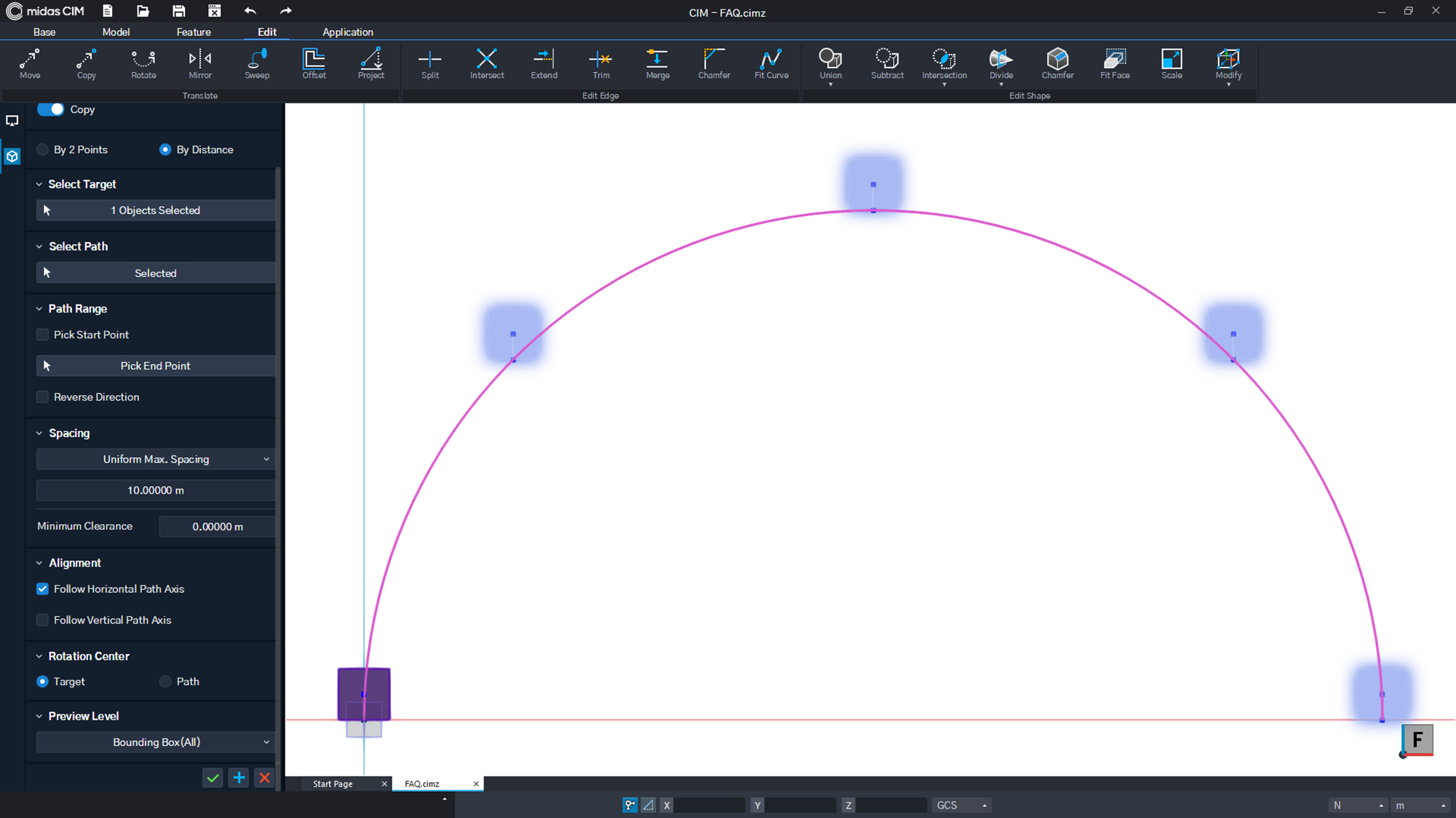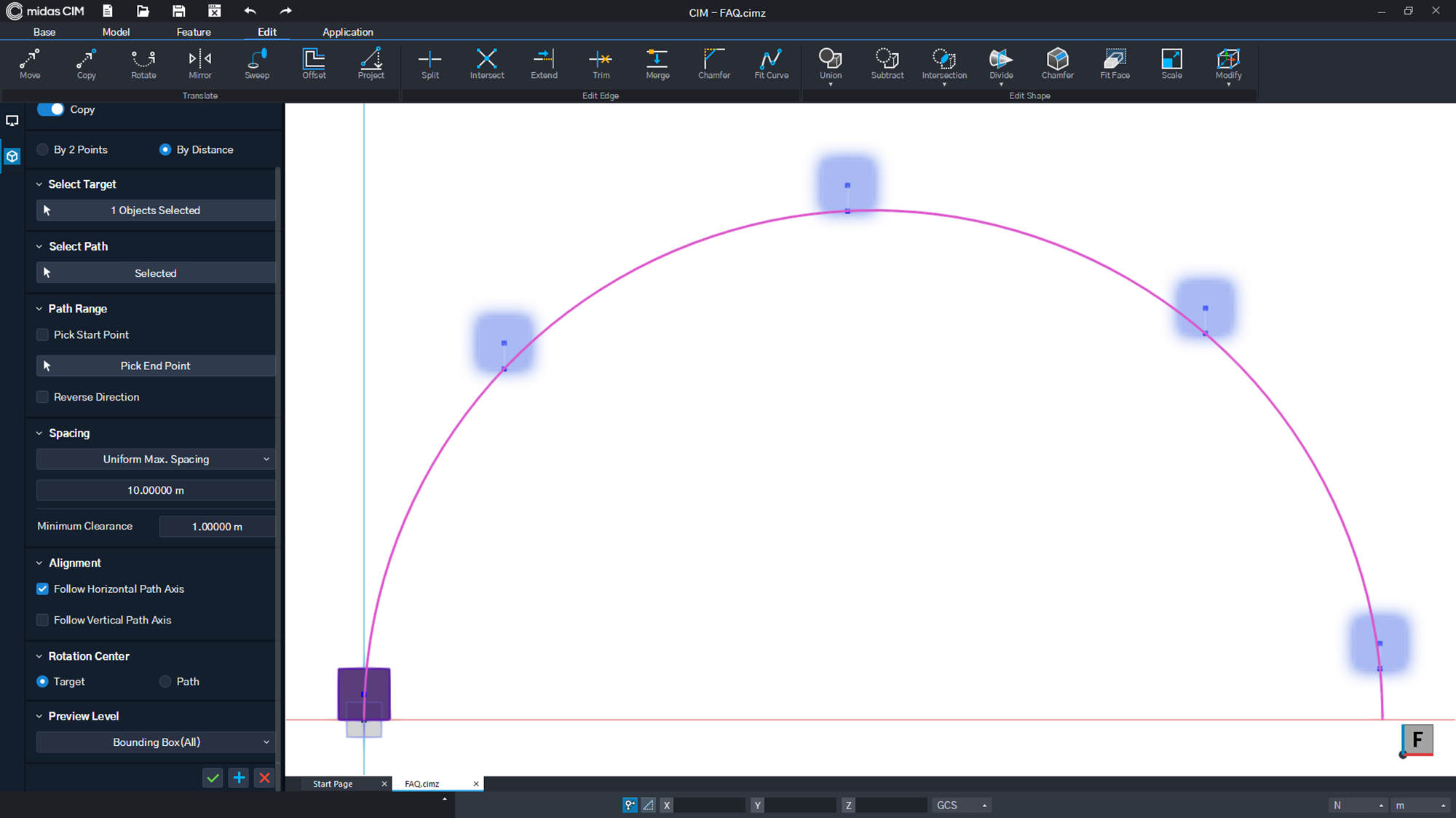Figure. 5 Uniform Max. Spacing (Spacing=10.0m) Figure. 6 Uniform Max. Spacing(Spacing=10.0m, Min. Clearance=1.0m)

Uniform Min. Spacing
ㆍThe target object (Solid) is copied at an interval larger than the Uniform Max.
Spacing of 10m along the defined arc path (R=10.0m) (Figure. 7)
ㆍSince the circumference of the semi-circle is πr=31.4m, the target object will be copied three times when a value
larger than 10m is applied. (Figure. 8)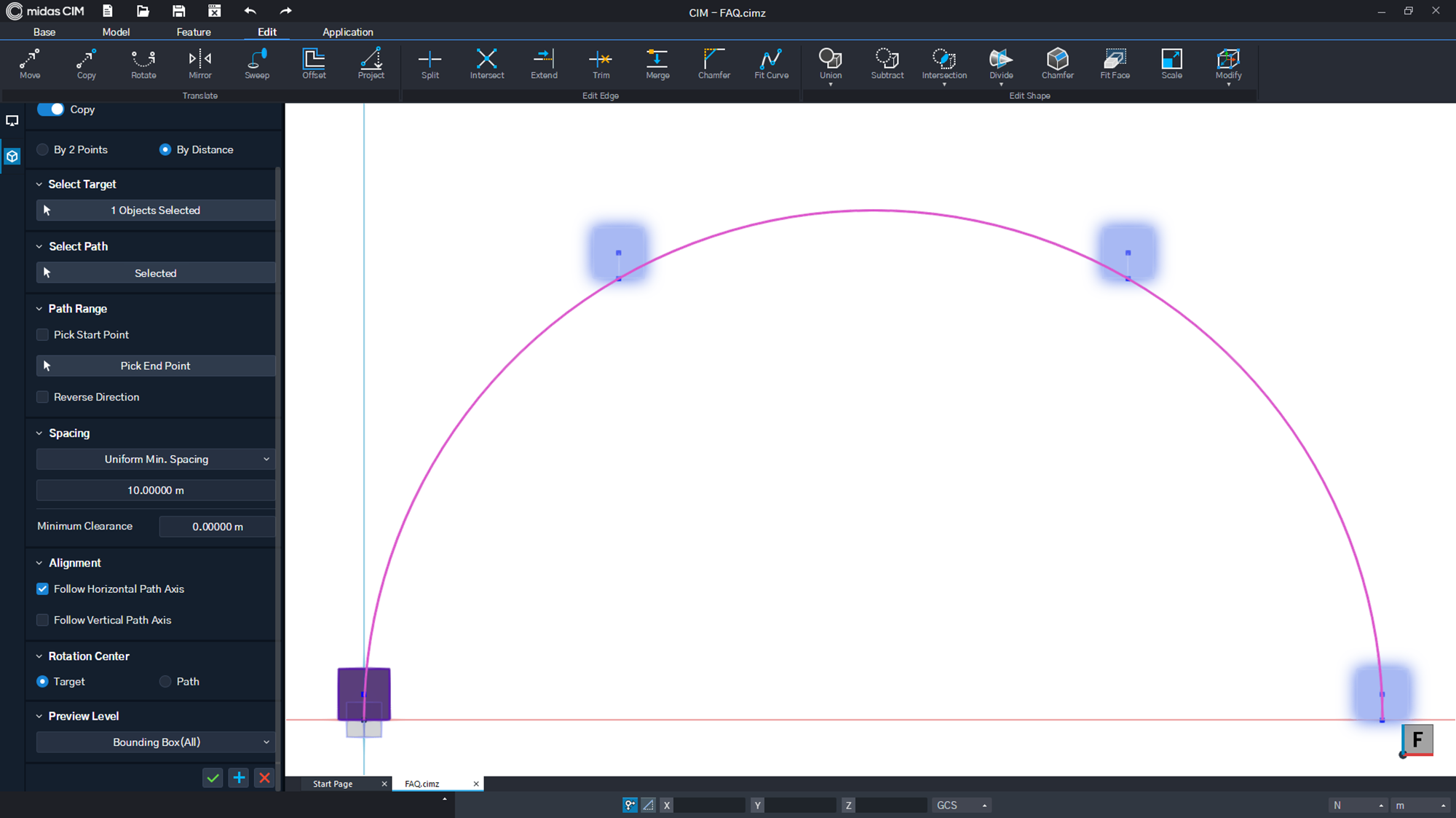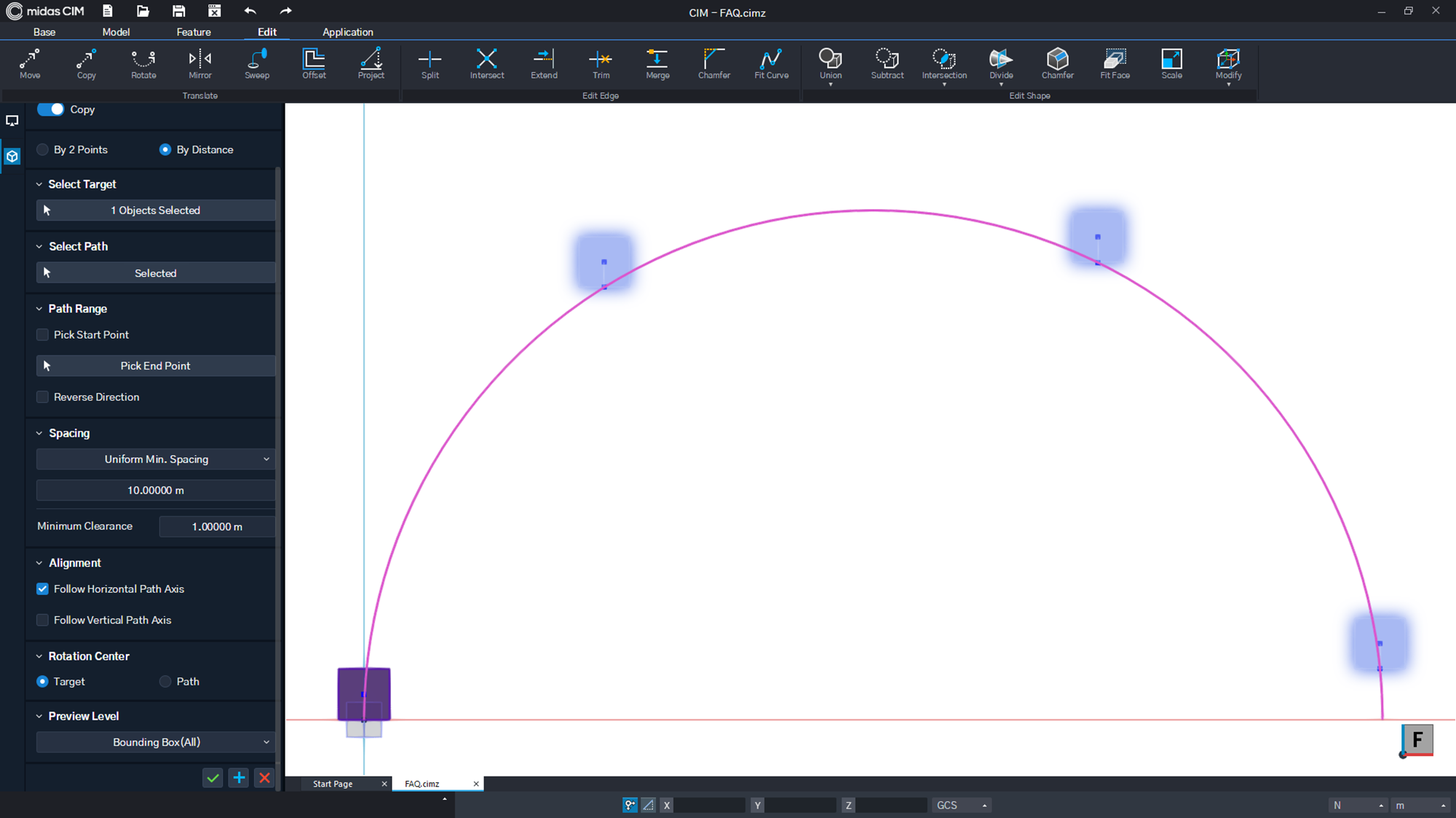Figure. 7 Uniform Min. Spacing (Spacing=10.0m) Figure. 8 Uniform Min. Spacing(Spacing=10.0m, Min. Clearance=1.0m)

Alignment
ㆍFollow Horizontal Path Axis : When checked, it is defined as the local coordinate system of the path based on the
object’s horizontal direction.(Default : Check on) (Figure. 9)
ㆍFollow Vertical Path Axis : When checked, it is defined as the local coordinate system of the path based on the
object’s vertical direction. (Figure. 10)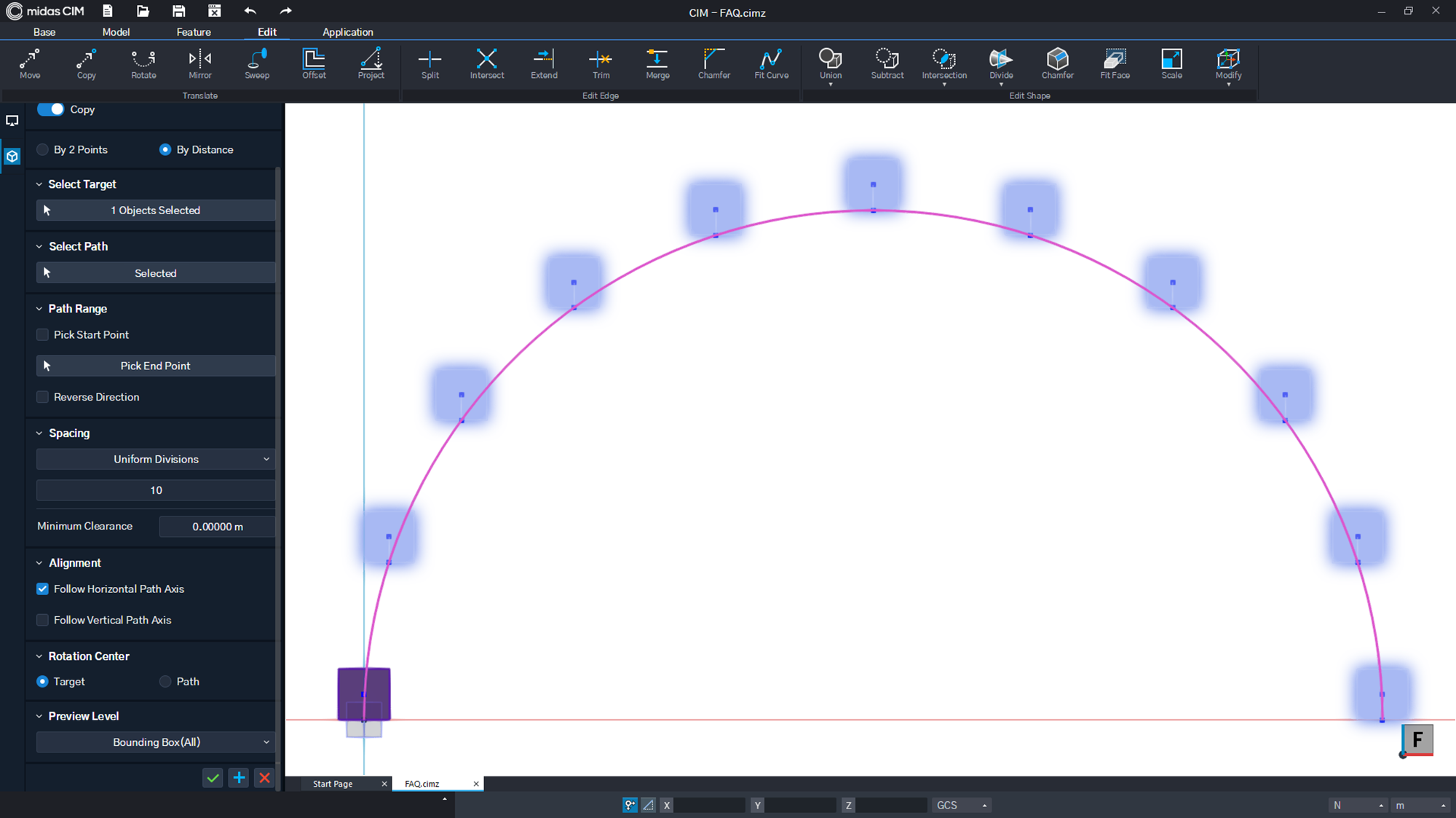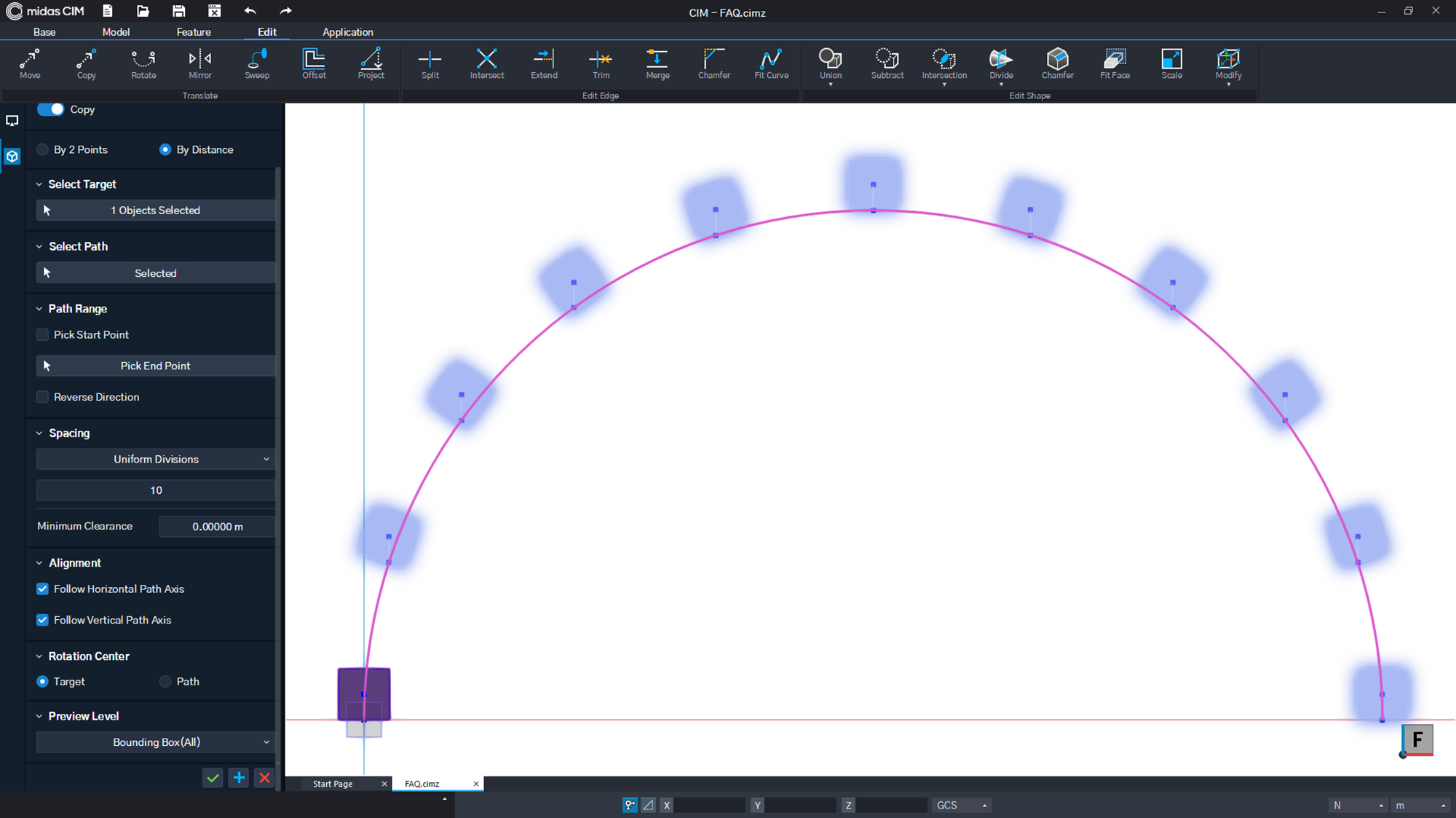Figure. 9 Follow Horizontal Path Axis (Check On) Figure. 10 Follow Vertical Path Axis (Check On)
0